# The Triaugmented Truncated Dodecahedron

The triaugmented truncated dodecahedron is the 71st Johnson solid, or J71 for short. It has 62 faces (9 decagons, 3 pentagons, 15 squares, and 35 triangles), 135 edges, and 75 vertices. It is obtained by augmenting three non-adjacent decagonal faces of the truncated dodecahedron with pentagonal cupolae (J5).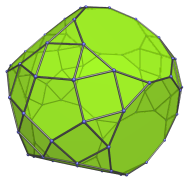If one of the augments is removed, the metabiaugmented truncated dodecahedron (J70) is produced. If two augments are removed, what remains is the augmented truncated dodecahedron (J68).

## Projections

Here are some views of the triaugmented truncated dodecahedron from various angles:

Projection Description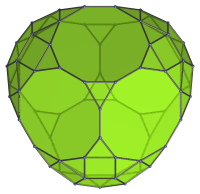Top view.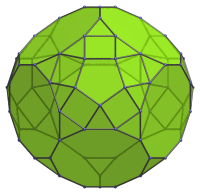Front view, parallel to pair of opposite triangles.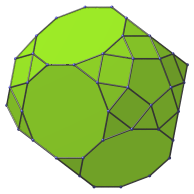Side view.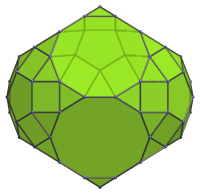Oblique view centered on decagon-decagon edge.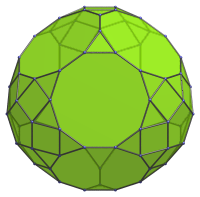Centered on decagon.

## Coordinates

The Cartesian coordinates of the triaugmented truncated dodecahedron with edge length 2 are even permutations of coordinate and all changes of sign of:

• (1, 0, 1+3φ)
• 2, 1, 2φ2)
• (2φ, φ2, φ3)

along with:

• (±(13φ+1)/5, ±1, (6φ+12)/5)
• (±(13φ+6)/5, ±φ, (φ+12)/5)
• (±(18φ+1)/5, 0, (φ+7)/5)
• (±1, −(6φ+12)/5, −(13φ+1)/5)
• (±φ, −(φ+12)/5, −(13φ+6)/5)
• (0, −(φ+7)/5, −(18φ+1)/5)

where φ=(1+√5)/2 is the Golden Ratio.

Last updated 27 Apr 2020.